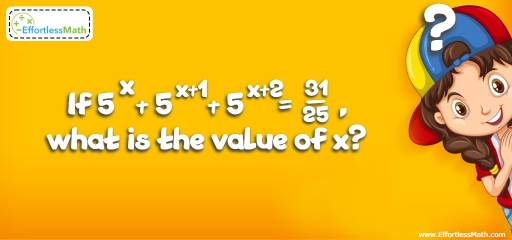# Ratio, Proportion and Percentages Puzzle -Critical Thinking 8

This kind of math puzzles is an educational activity for practicing children's math skills. It's also helpful for adults to have fun and challenge their brains!## Challenge:

If $$5^x + 5^{x+1} + 5^{x+2}=\frac{31}{25}$$, what is the value of x?

### The Absolute Best Book to challenge your Smart Student!

The correct answer is $$-2$$.

Solve for x:
$$5^x + 5^{x+1} + 5^{x+2}=\frac{31}{25}$$
The common factor is $$5^x$$. Then,
$$5^x(1+5^1+5^2)=\frac{31}{25}⇒5^x(31)=\frac{31}{25}⇒5^x=\frac{\frac{31}{25}}{31}⇒$$
$$5^x=\frac{31}{25}÷\frac{31}{1}=\frac{31}{25}×\frac{1}{31}=\frac{1}{25}$$
$$\frac{1}{25}=5^{-2}$$ Then: $$5^x=5^{-2}⇒ x = -2$$

### What people say about "Ratio, Proportion and Percentages Puzzle -Critical Thinking 8 - Effortless Math: We Help Students Learn to LOVE Mathematics"?

No one replied yet.

X
30% OFF

Limited time only!

Save Over 30%

SAVE $5 It was$16.99 now it is \$11.99